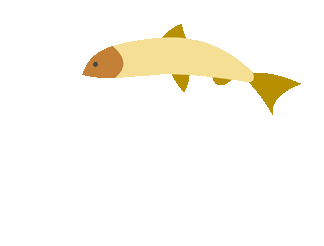top of page## Multiplication Games

##### Whole Number Multiplication (Games)
###### Multiplication with 1 and 2-Digit Multipliers (Games)
1. Math Playground

Use the product line boxes to multiply and help you find the final product.

2. AAA Math
Scroll down to the bottom to solve whole number multiplication problems.

3. Baseball Multiplication
See if you can avoid getting three strikes, by answering the question correctly on the first try.

4. Math Playground
See if you can win the canoe race by answering the questions correctly.

##### Decimal Multiplication (Resources)
###### Decimal Multiplication with 1 and 2-Digit Multipliers (Resources)
1. AAA Math: Multiplication Lessons

Online lessons on multiplication.

2. Instructional help with exponents.

3. In depth notes, examples, and practice with multiplying decimals.

4. Detailed notes and examples.

##### Decimal Multiplication (Games)

Multiplication with 1 and 2-Digit Multipliers

1. Multiplication Millionaire

Answer multiplication questions to reach the million dollar questions

2. Soccer Shoot Out

Practice two digit multiplication with whole numbers.

###### Multiplication with Decimals
1. Decimals Jeopardy

Review adding and subtracting decimals and practice multiplying decimals.

2. Decimal Cruncher

See how well you do!

3. Multiply Whole Numbers by Decimals Numbers

##### Exponent (Resources)
1. Math Goodies

2. Practice Makes Prefect

Test your knowledge and get instant feedback!

3. Math.Com Exponents

In depth notes, examples, and practice with exponents.

##### Exponents (Games)
1. Lots of Different Games

Choose from a multitude of different exponents games.

2. Battleship Exponents

Play battleship and practice with exponents.

##### Multiplying By Powers of 10 (Games)
1. Quia Quiz
Matching Game

2. Multiplying by Powers of 10
Practice Understanding Patterns with Zeros when MUltiplying Exponents

3. Mr. Nussbaum
Test your knowledge with the quiz.

## Timestables.combottom of page